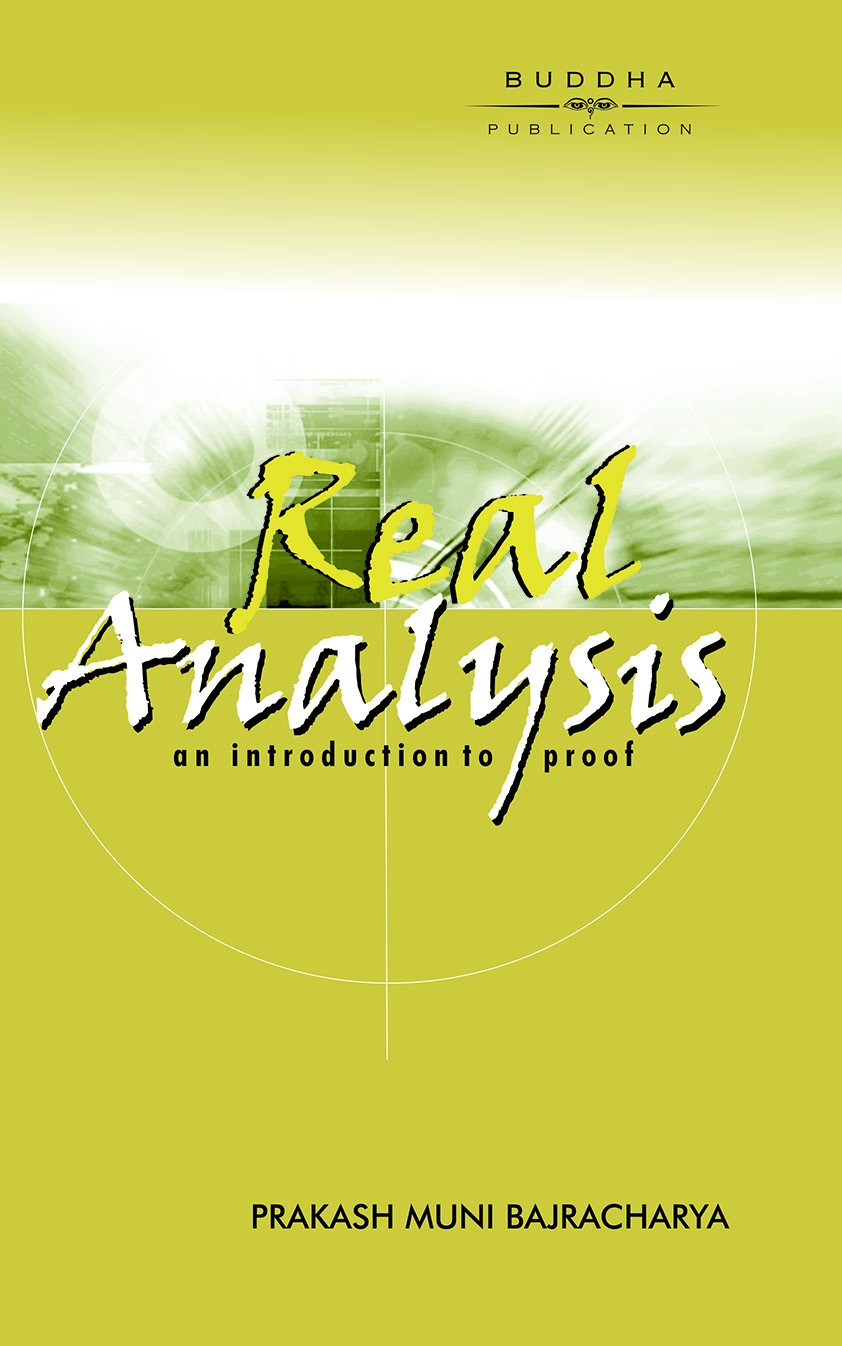## Real Analysis: An Introduction To Proof

Author: Prof. Dr. Prakash Muni Bajracharya
• ISBN: 9789937301831
• Version: English
• Price NRs. 365
• Avaliablility: In stock
• University:

This book covers the material contained in the course "Mathematical Analysis I" (Math 322) prescribed by Tribhuvan University for B.Sc./B.A. In the book, an attempt is made to provide some insight into a mathematical concept by first discussing the intuitive idea and then expressing the idea mathematically. Any new concept has been introduced only when it is needed in the logical development of the topic. The main aim of the book is to help the student in developing his/her ability of mathematical reasoning. The study of the proofs helps the student to acquire practice in logical argument and provides prerequisites for further mathematical education.
In the book the student will learn enough concepts, theorems, proof techniques and mathematical language in general to be well prepared for the further study in mathematical analysis and in modern mathematics, in general. However, a student's first encounter with analysis has been widely regarded as the most difficult course in the B.Sc./B.A. mathematics curriculum. This is not due so much to the complexity of the subject matter as it is to what the student is asked to do with it. After years of emphasizing computation, the student is now expected to be able to read, understand and actually construct mathematical proofs. Unfortunately, there is often very little groundwork laid to explain the nature and techniques of proofs.
The material is organized into nine chapters. To provide some helps for the students in their analyzing and constructing mathematical proofs, Chapter 1 takes a careful look at the rules and laws of logic and then examines how these rules and laws are used in the structuring of mathematical proofs by means of a discussion of basic types of proofs.
Chapter 2 provides an intuitive discussion of sets and functions followed by a briefing of cardinality.
Chapter 3 introduces the real number system axiomatically. A considerable amount of attention is given to the concepts of supremum and infimum and to the consequences of the completeness axiom.
Chapter 4 provides the basic topological structure of the real number system by introducing most of the modern terminology of metric spaces, although there is no separate chapter in metric spaces.
In Chapter 5, a thorough treatment of sequences of real numbers and the associated limit concept is presented. The nested intervals theorem with the proof based on convergent sequences is given at the end of the chapter.
In Chapter 6, the convergence properties of sequences are applied to infinite series of real numbers. Several useful tests for convergence are discussed as well.
Chapter 7 deals with the notion of a limit of a function playing a fundamental role in mathematical analysis. This chapter introduces continuity of a function and provides its relationship both to limits of functions and to sequences. Some important properties of continuity are developed and uniform continuity is discussed, as well.
Chapter 8 is devoted to an important process: differentiation. The focus of this chapter is on the theoretical aspects of differentiation that are often treated more superficially in the calculus course. It also includes Taylor's polynomial with several forms of a remainder.
Chapter 9 explores the theory of Riemann integration with a discussion of an alternative approach.
Almost all the material in the book has been organized from the lecture notes given by the author to B.Sc./B.A. students at different colleges during several years. Throughout the book, attention has been given to making the text accessible to students so that they can easily read and understand the text. There are numerous examples and figures that illustrate the concepts being discussed. These examples have been carefully chosen to develop the student's understanding, not just to illustrate a concept.